## Tamilnadu Samacheer Kalvi 12th Maths Solutions Chapter 12 Discrete Mathematics Ex 12.1

Question 1.
Determine whether * is a binary operation on the sets given below
(i) a * b = a.|b| on R ,
(ii) a * b = min (a, b) on A = {1, 2, 3, 4, 5}
(iii) (a * b) = $$a \sqrt{b}$$ is binary on R.
Solution:
(i) Yes.
Reason: a, b ∈ R. So, |b| ∈ R when b ∈ R
Now multiplication is binary on R
So a|b| ∈ R when a,be R.
(Le.) a * b ∈ R.
* is a binary operation on R.

(ii) Yes.
Reason: a, b ∈ R and minimum of (a, b) is either a or b but a, b ∈ R.
So, min (a, b) ∈ R.
(Le.) a * b ∈ R.
* is a binary operation on R.

(iii) a* b = $$a \sqrt{b}$$ where a, b ∈ R.
No. * is not a binary operation on R.
Reason: a, b ∈ R.
⇒ b can be -ve number also and square root of a negative number is not real.
So $$\sqrt{b}$$ ∉ R even when b ∈ R.
So $$\sqrt{b}$$ ∉ R. ie., a * b ∉ R.
* is not a binary operation on R.Question 2.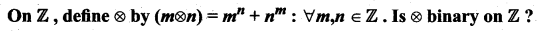Solution:
No. * is not a binary operation on Z.
Reason: Since m, n ∈ Z.
So, m, n can be negative also.
Now, if n is negative (Le.) say n = -k where k is +ve.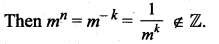Similarly, when m is negative then nm ∉ Z.
∴ m * n ∉ Z. ⇒ * is not a binary operation on Z.

Question 3.
Let * be defined on R by (a * b) = a + b + ab – 1 .Is * binary on R ? If so, find $$3 *\left(\frac{-7}{15}\right)$$
Solution:
a * b = a + b + ab – 7.
Now when a, b ∈ R, then ab ∈ R also a + b ∈ R.
So, a + b + ab ∈ R.
We know – 7 ∈ R.
So, a + b + ab – 7 ∈ R.
(ie.) a * b ∈ R.
So, * is a binary operation on R.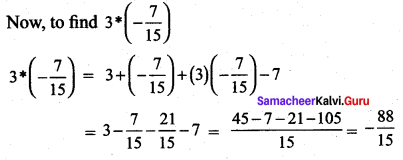Question 4.
Let A= {a+ $$\sqrt{5}$$b: a, b ∈ Z}. Check whether the usual multiplication is a binary operation on A.
Solution:
Let A = a + $$\sqrt{5}$$ b and B = c + $$\sqrt{5}$$d, where a, b, c, d ∈ M.
Now A * B ={a + $$\sqrt{5}$$b)(c + $$\sqrt{5}$$d)
= ac + $$\sqrt{5}$$ad + $$\sqrt{5}$$bc + $$\sqrt{5}$$b$$\sqrt{5}$$d
= (ac + 5bd) + $$\sqrt{5}$$(ad+ bc) ∈ A
Where a, b, c, d ∈ Z
So * is a binary operation.Question 5.
(i) Define an operation * on Q as follows: a * b = $$\left(\frac{a+b}{2}\right)$$; a, b ∈ Q. Examine the closure,
commutative, and associative properties satisfied by * on Q.
(ii) Define an operation * on Q as follows: a*b = $$\left(\frac{a+b}{2}\right)$$; a, b ∈ Q. Examine the existence of identity and the existence of inverse for the operation * on Q.
Solution:
(i) 1. Closure property:
Let a,b ∈ Q.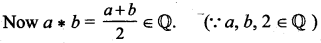So, closure property is satisfied.

2. Commutative property:
Let a, b ∈ Q.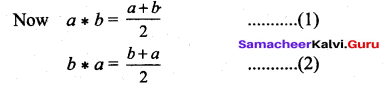(1) = (2) ⇒ Now a * b = b * a
⇒ Commutative property is satisfied.

3. Associative property:
Let a,b,c G Q. ^
To prove associative property we have to prove that a * (b * c) = (a * b) * c
LHS: a * (b * c)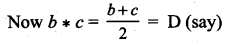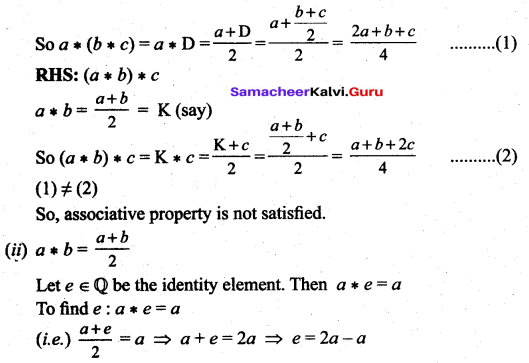(i.e.) the identity Clement e = a which is not possible.
So, the identity element does not exist and so inverse does not exist.Question 6.
Fill In the following table so that the binary operation * on A = {a, b, c} is commutative.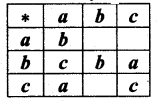Solution:
Given that the binary operation * is Commutative.
To find a * b :
a * b = b * a (∵ * is a Commutative)
Here b * a = c. So a * b = c
To find a *c: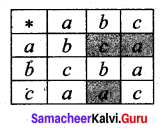a * c = c * a (∵ * is a Commutative)
c * a = a. (Given)
So a * c = a
To find c * b:
c * b = b * c
Here b * c = a.
So c * b = a

Question 7.
Consider the binary operation * defined on the set A = [a, b, c, d] by the following table: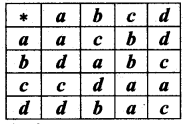– Is it commutative and associative?
Solution:
From the table
b * c = b
c * b = d
So, the binary operation is not commutative.
To check whether the given operation is associative.
Let a, b, c ∈ A.
To prove the associative property we have to prove that a * (b * c) = (a * b) * c
From the table,
LHS: b * c = b
So, a * (b * c) = a * b = c ……. (1)
RHS: a * b = c
So, (a * b) * c = c * c = a …… (2)
(1) ≠ (2). So, a * (b * c) ≠ (a * b) * c
∴ The binary operation is not associative.

Question 8.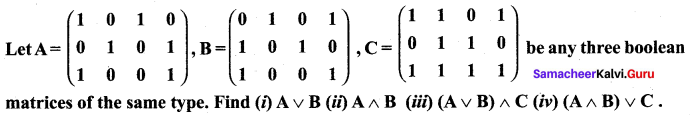Solution: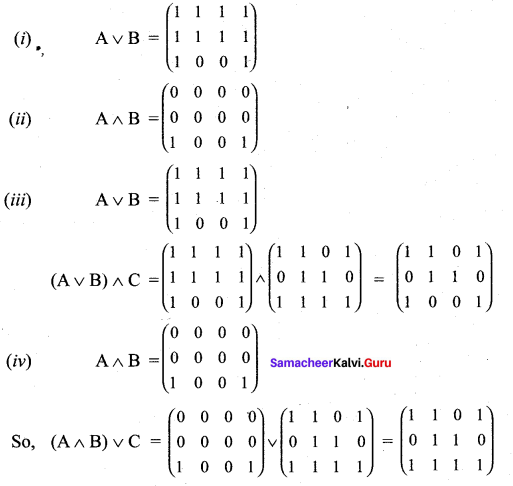Question 9.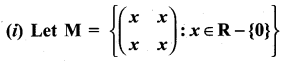and Let * be the matrix multiplication. Determine whether M is closed under * . If so, examine the commutative and associative properties satisfied by * on M .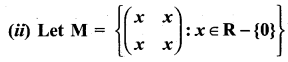and let * be the matrix multiplication. Determine whether M is closed under *. If so, examine the existence of identity, existence of inverse properties for the operation * on M.
Solution: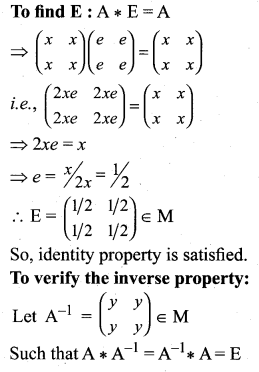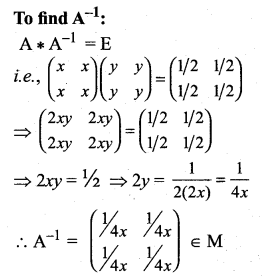So, inverse property is satisfied.Question 10.
(i) Let A be Q\{1). Define * on A by x * y = x + y – xy. Is * binary on A ? If so, examine the commutative and associative properties satisfied by * on A.
(ii) Let A be Q\{1}. Define *on A by x * y = x + y – xy. Is * binary on A ? If so, examine the existence of identity, existence of inverse properties for the operation * on A.
Solution:
(i) Let a,b ∈ A (i.e.) a ≠ ±1 , b ≠ 1
Now a * b = a + b – ab
If a + b – ab = 1 ⇒ a + b – ab – 1 = 0
(i.e.) a(1 – b) – 1(1 – b) = 0
(a – 1)(1 – b) = 0 ⇒ a = 1, b = 1
But a ≠ 1 , b ≠ 1
So (a – 1) (1 – 6) ≠ 1
(i.e.) a * b ∈ A. So * is a binary on A.

To verify the commutative property:

Let a, b ∈ A (i.e.) a ≠ 1 , b ≠ 1
Now a * b = a + b – ab
and b * a = b + a – ba
So a * b = b * a ⇒ * is commutative on A.

To verify the associative property:
Let a, b, c ∈ A (i.e.) a, b, c ≠ 1
To prove the associative property we have to prove that
a * (b * c) = (a * b) * c

LHS: b * c = b + c – bc = D(say)
So a * (b * c) = a * D = a + D – aD
= a + (b + c – bc) – a(b + c – bc)
= a + b + c – bc – ab – ac + abc
= a + b + c – ab – bc – ac + abc …… (1)

RHS: (a * b) = a + b – ab = K(say)
So (a * b) * c = K * c = K + c – Kc
= (a + b – ab) + c – (a + b – ab) c
= a + b – ab + c – ac – bc + abc
= a + b + c – ab – bc – ac + abc ….. (2)

(ii) To verify the identity property:
Let a ∈ A (a ≠ 1)
If possible let e ∈ A such that
a * e = e * a = a
To find e:
a * e = a
(i.e.) a + e – ae = a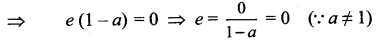So, e = (≠ 1) ∈ A
(i.e.) Identity property is verified.
To verify the inverse property:
Let a ∈ A (i.e. a ≠ 1)
If possible let a’ ∈ A such that
To find a’:
a * a’ = e
(i.e.) a + a’ – aa’ = 0
⇒ a'(1 – a) = – a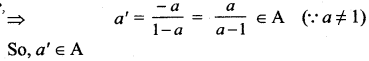⇒ For every a ∈ A there is an inverse a’ ∈ A such that
a* a’ = a’ * a = e
⇒ Inverse property is verified.### Samacheer Kalvi 12th Maths Solutions Chapter 12 Discrete Mathematics Ex 12.1 Additional Problems

Question 1.
Show that the set G = {a + b$$\sqrt{2}$$/ a, b ∈ Q} is an infinite abelian group with respect to Binary operation addition. Satisfies closure, associative, identity and inverse properties.
Solution:
(i) Closure axiom :
Let x, y ∈ G. Then x = a + b$$\sqrt{2}$$, y = c + d$$\sqrt{2}$$; a, b, c, d ∈ Q.
x + y = (a + b$$\sqrt{2}$$) + (c + d$$\sqrt{2}$$) = (a + c) + (b + d) $$\sqrt{2}$$ ∈ G,
Since (a + c) and (b + d) are rational numbers.
∴ G is closed with respect to addition.

(ii) Associative axiom : Since the elements of G are all real numbers, addition is associative.

(iii) Identity axiom : There exists 0 = 0 + 0 $$\sqrt{2}$$ ∈ G
such that for all x = a + b$$\sqrt{2}$$ ∈ G.
x + 0 = (a + b$$\sqrt{2}$$ ) + (0 + 0$$\sqrt{2}$$)
= a + b$$\sqrt{2}$$ = x
Similarly, we have 0 + x = x. ∴ 0 is the identity element of G and satisfies the identity axiom.

(iv) Inverse axiom: For each x = a + b$$\sqrt{2}$$ ∈ G,
there exists -x = (-a) + (-b) $$\sqrt{2}$$ ∈ G
such that x + (-x) = (a + b$$\sqrt{2}$$) + ((-a) + (- b)$$\sqrt{2}$$)
= (a + (-a)) + (b + (-b)) $$\sqrt{2}$$ = 0
Similarly, we have (- x) + x = 0 .
∴ (- a) + (-b)$$\sqrt{2}$$ is the inverse of a + b $$\sqrt{2}$$ and satisfies the inverse axiom.

(v) Commutative axiom:
x + y = (a + c) + (b + d) $$\sqrt{2}$$ = (c + a) + (d + b) $$\sqrt{2}$$
= (c + d$$\sqrt{2}$$) + (a + b$$\sqrt{2}$$)
= y + x, for all x, y ∈ G.
∴ The commutative property is true.
∴ (G, +) is an abelian group. Since G is infinite, we see that (G, +) is an infinite abelian group.Question 2.
Show that (Z7 – { }, .7) write to the binary operation multiplication modul07 satisfies closure, associative, identity and inverse properties.
Solution:
Let G = [, ,… ]
The Cayley’s table is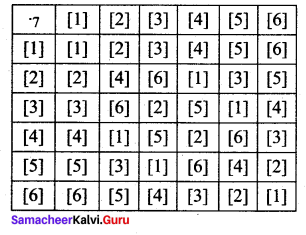From the table:
(i) all the elements of the composition table are the elements of G.
∴ The closure axiom is true.
(ii) multiplication modulo 7 is always associative.
(iii) the identity element is  ∈ G and satisfies the identity axiom.
(iv) the inverse of  is ;  is ;  is ;  is ;  is  and  is  and it satisfies the inverse axiom.

Question 3.
Show that the set G of all positive rationals with respect to composition * defined by ab
a* b = $$\frac{a b}{3}$$ for all a, b ∈ G satisfies closure, associative, identity and inverse properties.
Solution:
Let G = Set of all positive rational number and * is defined by,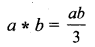(i) Closure axiom: Let a, b ∈ G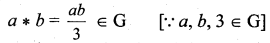∴ closure axiom is satisfied.

(ii) Associative axiom: Let a, b, c ∈ G.
To prove the associative property, we have to prove that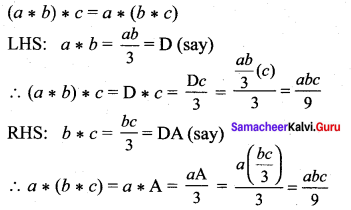(1) = (2) ⇒ LHS = RHS i.e., associative axiom is satisfied.

(iii) Identity axiom: Let a ∈ G.
Let e be the identity element.
By the definition, a * e = a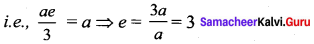e = 3 ∈ G ⇒ identity axiom is satisfied.(iv) Inverse axiom: Let a ∈ G and a’ be the inverse of a * a’ = e = 3.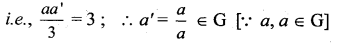Question 4.
Show that the set G of all rational numbers except – 1 satisfies closure, associative, identity and inverse property with respect to the operation * given by a * b = a + b + ab for all a, b ∈ G
Solution:
G = [Q, -{-l}]
* is defined by a * b = a + b + ab
To prove G is an abelian group.

G1: Closure axiom: Let a, b ∈ G.
i.e., a and b are rational numbers and a ≠ -1, b ≠ -1.
So, a * b = a + b + ab
If a + b + ab = – 1
⇒ a + b + ab + 1 = 0
i.e., (a + ab) + (b + 1) = 0
a (1 + b) + (b + 1) = 0
i.e., (a + 1)(1 + b) = 0
⇒ a = -1, b = -1
But a ≠ -1, b ≠ -1
⇒ a + b + ab ≠ -1
i.e., a + b + ab ∈ G ∀ a, b ∈ G
⇒ Closure axiom is verified.

G2: Associative axiom: Let a, b, c ∈ G.
To prove G2, we have to prove that,
a * {b * c) = (a * b) * c
LHS:
b * c = b + c + bc = D (say)
a * (b * c) = a * D = a + D + aD
= a + (b + c + bc) + a(b + c + bc)
= a + b + c + bc + ab + ac + abc
= a + b + c + ab + bc + ac + abc ……. (1)
RHS:
a * b = a + b + ab = E (say)
∴ (a * b) * c = E * c = E + c + Ec
= a + b + ab + c + (a + b + ab) c
= a + b + ab + c + ac + bc + abc ……. (2)
= a + b + c + ab +be+ ac + abc
(1) = (2) ⇒ Associative axiom is verified.

G3: Identity axiom: Let a ∈ G. To prove G3 we have to prove that there exists an element e ∈ G such that a * e = e * a = a.
To find e: a * e = a
i.e., a + e + ae = a
⇒ e(1 + a) = a – a = 0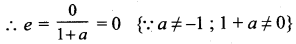So, e = 0 ∈ G ⇒ Identity axiom is verified.

G4 : Inverse axiom: Let a ∈ G. To prove G4, we have to prove that there exists an element a’ ∈ G such that a * a’ = a’ * a = e.
To find a’: a * a’ = e
i.e., a + a’ + aa’=: 0 {∵ e = 0}
⇒ a'(1 + a) = -a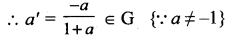Thus, inverse axiom is verified.Question 5.
Show that the set { , , , , } under multiplication modulo 11 satisfies closure, associative, identity and inverse properties.
Solution:
G = {, , , , }
* is defined by multiplication modulo 11.
To prove G is an abelian group with respect to *
Since we are given a finite number of elements i.e., since the given set is finite, we can frame the multiplication table called Cayley’s table.
The Cayle’s table is as follows: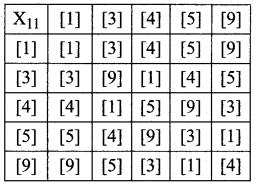G1: The elements in the above table are , , ,  and  which are elements of G.
∴ closure axiom is verified.

G2: Consider , ,  which are elements of G.
{ * } *  =  *  =  ……. (1)
 * { * } =  *  =  …… (2)
(1) = (2) ⇒ (a * b) * c = a * (b * c) i.e., associative axiom is verified.

G3: The first row elements are the same as that of the given elements in the same order. ie., from the table, the identity element is  ∈ G. So identity axiom is verified.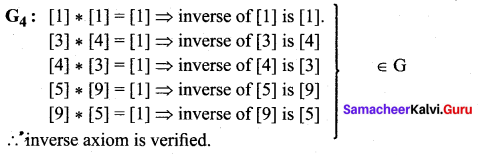G5: From the table * is commutative i.e., the entries equidistant from the leading diagonal on either sides are equal ⇒ a * b = b * a